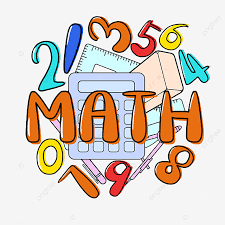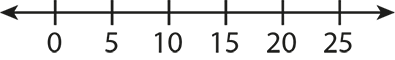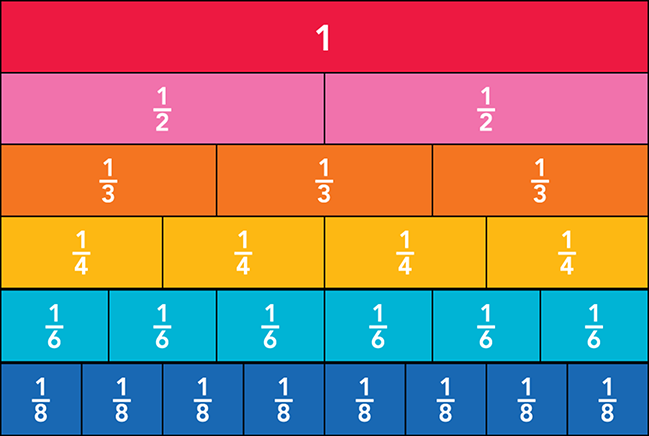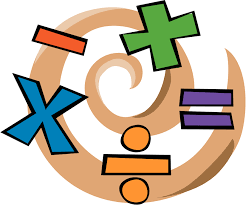# MATHCHAPTER 11- VOCABULARY

 fraction model For a fraction a/b, a figure divided into b equal parts with a of those parts shaded. improper fraction A fraction with a numerator that is greater than or equal to its denominator. Example: 5/3 unit fraction A fraction with a numerator of 1. numerator The numeral above the bar in a fraction; it names the number of parts being considered. Example:like denominators Denominators that are the same in two or more fractions. decompose To break into smaller parts or groups. number line A line that shows numbers in order using a scale.addend A number that is added to another number or numbers. mixed number A number made up of a whole number part and a fraction part. Example: 3 1/2 fraction strips A rectangular strip divided into equal parts, where each part represents a unit fraction.Chapter 11 Topics
 Lesson 11-1: Use Models to Add Fractions Lesson 11-2: Add Fractions: Like Denominators Lesson 11-3: Decompose Fractions as Sums of Unit Fractions Lesson 11-4: Use Models to Subtract Fractions Lesson 11-5: Subtract Fractions: Like Denominators Lesson 11-6: Write Mixed Numbers as Equivalent... Lesson 11-7: Add Mixed Numbers: Like Denominators Lesson 11-8: Subtract Mixed Numbers: Like Denominators Lesson 11-9: Problem Solving: More Than One Way

WHAT TO EXPECT...

Students can expect to be ASSESSED in the following ways:

Classwork/Homework
Fluency tests
Quizzes
Tests
Projects

MATH FACT FLUENCY

• I can't stress this enough, MASTERY is expected with all basic facts!
• Please make sure your child is studying their math facts. Knowing their math facts will strengthen their skills and help them understand all the new concepts they will be learning.
• Plan to practice a minimum of 3 times a week for 10 minutes a session (on-line sites and/or games).

SplashLearn
This school year I plan to use an educational program called SplashLearn to supplement their math learning.  We will utilize this program in school, but I may give additional assignments that students can work on at home.  The version we will be using will give us access to assigned practice, math facts, and progress reports.Websites:
A-Plus Math
Multiplication
Math Playground
Cool Math for Kids
The Kids Pages
Fun Brain